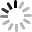# SVG 研究之路 (26) - 有趣的 Patterns

Patterns 是 SVG fill 裏頭的一個有趣元素，記得在 CSS 裏頭，我們可以自訂背景的圖案，達到許多很有特色的變化，然而在 SVG 也是如此，而 SVG 比 CSS 更加強大的地方，是你所有的圖案幾乎都可以在 SVG 裏頭畫出來 ( 當然強調細節的點陣圖案例外 )，以下將介紹 Patterns 的相關屬性與參數設定，並直接來看一些 Patterns 的範例。

• patternUnits = "userSpaceOnUse | objectBoundingBox"
• patternContentUnits = "userSpaceOnUse | objectBoundingBox"
• patternTransform
• x
• y
• width
• height
• preserveAspectRatio = "[defer] []"

## patternUnits = "userSpaceOnUse | objectBoundingBox"

patternUnits，這個屬性非常的奇怪，因為這個屬性有兩個參數可以選，而這兩個參數所對應後續的 width 和 height 是不同的，也因此非常容易混淆，如果將 patternUnits 設定為`userSpaceOnUse`，表示以使用者的座標為主，後面的 width 和 height 就是實際的寬和高，例如下面的範例，SVG 的寬高是 240x160，我們將 pattern 的寬高都設為 60，表示我們將在 240x160 的範圍內重複填滿 60x60 的 pattern ( 也就是圖中的藍色框框內的 )，同時我們也可以發現，不管我們的圖形放在哪，圖案都會以 SVG 的左上角 0,0 為基準點 ( 因為內容 pattern 的 rect 設為 0,0 )，可以看到綠色框的圓形，填滿的圖形和底下的圖形位置一模一樣。

``````<svg width="240" height="160">
<defs>
<pattern id="p" patternUnits="userSpaceOnUse" width="60" height="60">
<rect width="30" height="30" fill="#f99" x="0" y="0"></rect>
</pattern>
</defs>
<rect width="240" height="160" stroke="#aaa" fill="url(#p)" />
<circle cx="150" cy="80" r="50" stroke="#000" fill="url(#p)" />
<rect width="60" height="60" x="1" y="1" stroke="#00f" stroke-width="2" fill="url(#p)" />
</svg>
````````````<svg width="240" height="160">
<defs>
<pattern id="p" patternUnits="userSpaceOnUse" width="40" height="40">
<rect width="30" height="30" fill="#f99" x="0" y="0"></rect>
</pattern>
</defs>
<rect width="240" height="160" stroke="#aaa" fill="url(#p)" />
<circle cx="150" cy="80" r="50" stroke="#0a0" stroke-width="2" fill="url(#p)" />
<rect width="40" height="40" x="1" y="1" stroke="#00f" stroke-width="2" fill="url(#p)" />
</svg>
````````````<defs>
<pattern id="p" patternUnits="userSpaceOnUse" width="40" height="40">
<rect width="30" height="30" fill="#f99" x="30" y="0"></rect>
</pattern>
</defs>
````````````<defs>
<pattern id="p" patternUnits="objectBoundingBox" width="1" height="1">
<rect width="30" height="30" fill="#f99" x="0" y="0"></rect>
</pattern>
</defs>
<circle cx="180" cy="80" r="50" stroke="#0a0" stroke-width="2" fill="url(#p)" />
<rect width="100" height="100" x="10" y="30" stroke="#000" stroke-width="2" fill="url(#p)" />
````````````<defs>
<pattern id="p" patternUnits="objectBoundingBox" width=".4" height=".4">
<rect width="30" height="30" fill="#f99" x="0" y="0"></rect>
</pattern>
</defs>
<circle cx="180" cy="80" r="50" stroke="#0a0" stroke-width="2" fill="url(#p)" />
<rect width="100" height="100" x="10" y="30" stroke="#000" stroke-width="2" fill="url(#p)" />
````````````<defs>
<pattern id="p" patternUnits="objectBoundingBox" width=".4" height=".4">
<rect width="30" height="30" fill="#f99" x="0" y="0"></rect>
</pattern>
</defs><rect width="80" height="80" x="120" y="30" stroke="#000" stroke-width="2" fill="url(#p)" />
<rect width="100" height="100" x="10" y="30" stroke="#000" stroke-width="2" fill="url(#p)" />
``````## patternContentUnits = "userSpaceOnUse | objectBoundingBox"

patternContentUnits 跟剛剛提到的 patternUnits 幾乎是異曲同工，只是這個屬性是用來定義 pattern 內容畫出來的圖形單位，同樣的，設定為`userSpaceOnUse`代表是實際的寬度或高度數值，設定為`objectBoundingBox`的則是比例，但是！又是非常重要的但是！但是它的預設值為`userSpaceOnUse`，整個擺明整人呀！

``````<defs>
<pattern id="p1" patternUnits="objectBoundingBox" width=".4" height=".4" patternContentUnits="userSpaceOnUse">
<rect width="20" height="20" fill="#069" x="0" y="0"></rect>
</pattern>
<pattern id="p2" patternUnits="objectBoundingBox" width=".4" height=".4" patternContentUnits="objectBoundingBox">
<rect width=".2" height=".2" fill="#069" x="0" y="0"></rect>
</pattern>
</defs>

<rect width="100" height="100" x="120" y="30" stroke="#000" stroke-width="2" fill="url(#p1)" />
<rect width="100" height="100" x="10" y="30" stroke="#000" stroke-width="2" fill="url(#p2)" />
``````## patternTransform

``````<defs>
<pattern id="p1" patternUnits="objectBoundingBox" width=".2" height=".4" patternTransform="rotate(45)">
<rect width="10" height="50" fill="#000" x="0" y="0"></rect>
<rect width="10" height="50" fill="#fa0" x="10" y="0"></rect>
</pattern>
</defs>

<rect width="100" height="100" x="10" y="30" stroke="#000" stroke-width="2" fill="url(#p1)" />
``````## x,y,width,height

``````<defs>
<pattern id="r1">
<rect width="20" height="20" fill="#0c0" x="0" y="0"></rect>
</pattern>
<pattern id="p1" patternUnits="objectBoundingBox" width=".4" height=".4" xlink:href="#r1" />
<pattern id="p2" patternUnits="objectBoundingBox" width=".25" height=".25" xlink:href="#r1" />
</defs>

<rect width="100" height="100" x="10" y="30" stroke="#000" stroke-width="2" fill="url(#p1)" />
<rect width="100" height="100" x="120" y="30" stroke="#000" stroke-width="2" fill="url(#p2)" />
``````## preserveAspectRatio = "[defer] []"

``````<defs>
<pattern id="p1" patternUnits="objectBoundingBox" width=".4" height=".4" viewbox="0,0,22,22">
<rect width="20" height="20" fill="#f80" x="0" y="0"></rect>
</pattern>
<pattern id="p2" patternUnits="objectBoundingBox" width=".4" height=".4" viewbox="0,0,40,40">
<rect width="20" height="20" fill="#f80" x="0" y="0"></rect>
</pattern>
</defs>

<rect width="100" height="100" x="10" y="30" stroke="#000" stroke-width="2" fill="url(#p1)" />
<rect width="100" height="100" x="120" y="30" stroke="#000" stroke-width="2" fill="url(#p2)" />
``````